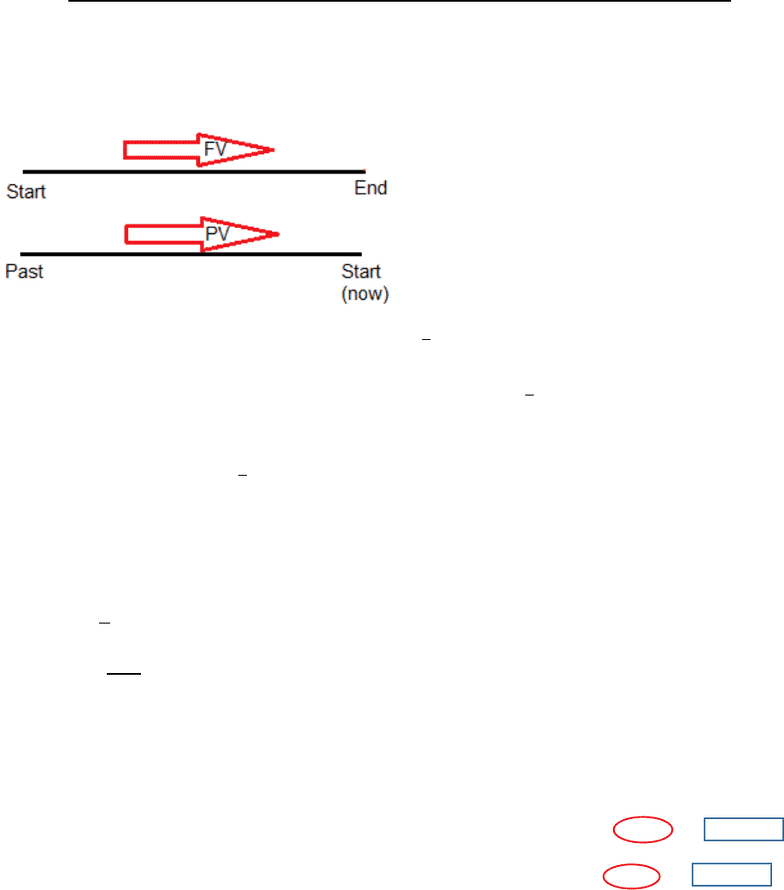Class Notes (1,100,000)
CA (630,000)
UTSC (30,000)
Mathematics (1,000)
MATA32H3 (200)
Lecture 3

# MATA32 Lecture 3: Present Value, Future Value, and Equations of ValuePremium

Department
Mathematics
Course Code
MATA32H3
Professor
Raymond Grinnell
Lecture
3

This preview shows half of the first page. to view the full 3 pages of the document.MATA 32 Lecture 3 Present Value, Future Value, and Equation of Value
Present and Future Value (5.2):
FV: If = principal (now), then   

o  is the future value of an investment of now
PV: If = compounded amount (now) then   

o  is the present (or past) value of an amount now
We can combine PV and FV into 1equation
o   

Example:
In 5 years we need \$35000 in an account. Interest is compounded weekly at 4.3% APR. What is
the present amount needed to be invested?
  

  
  ; where         (All in units of \$1000)

Therefore, \$28,231.46 must be invested now to reach \$35000 in 5 years.
Equations of Value (p. 214):
We have a “long period of time” throughout which we have interest, debt, and payment
(financial transaction)
An Equation of Value (EoV) is an equation that describes interest, debt, and payment
and allows us to calculate these 3 factors at specific times